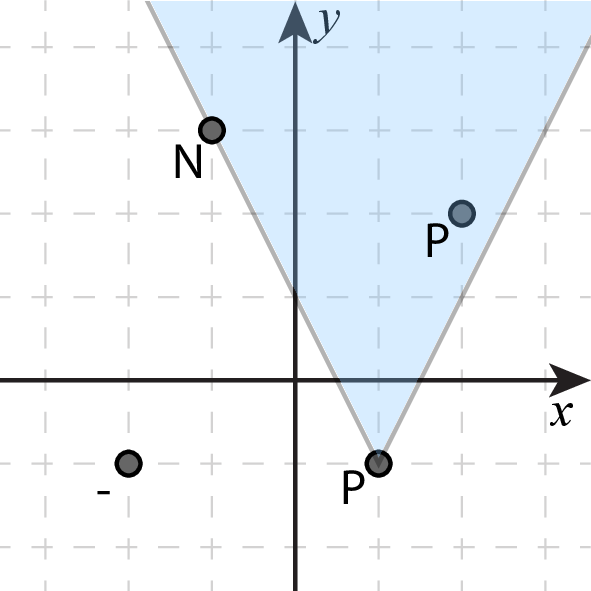시간 제한 메모리 제한 제출 정답 맞은 사람 정답 비율
2 초 1024 MB 0 0 0 0.000%

## 문제

You have been assigned to a new top-secret program involving a strange vacuum system. The physicists working on the system have been trying to find out where it is leaking but now they are confused by all the measurement results and want your help to figure out what is going on.

The vacuum system contains a wall with possibly leaking components. The physicists have performed vacuum leak tests on some of these components by flushing them with helium gas and then noting down whether their mass spectrometer detected any spike in helium in the vacuum system directly following this release of gas. If the component has even the tiniest leak they will detect it this way but there are some complications as well. The helium will rise up and spread out from where they released it and if it passes by any other leaking component, that will also trigger a positive reading. For each unit distance the helium has risen it will also have expanded by one unit. Thus the leak test will produce a positive result if the tested component is leaking or if there is a leaking component above it for which the $x$ coordinates differs by at most half of the difference in the $y$ coordinate.  See Figure 1 for an example.

You start out with a positive mindset thinking that there are probably just a few leaking components responsible for all the positive measurements. To determine if this is indeed possible you set out to determine the minimum number of leaking components that could give rise to the observed leak test results.Figure 1: Illustration of Sample Input 1. Circles indicate components and the blue triangle indicates where helium will spread when the first component is tested. This test being positive means that at least one of the the three components covered by the triangle is leaking. The correct answer in this case is 1 since the measurement results can all be explained with only the rightmost component leaking.

## 입력

The first line of input contains an integer $n$ ($1 \le n \le 2 \cdot 10^5$), the number of components involved. The following $n$ lines each contain two integers $x$ and $y$ and a character $c$ ($-10^8 \le x,y \le 10^8$, $c \in \{-, P, N\}$), where $(x, y)$ are the coordinates of a component and $c$ describes a possible leak test result, with the following meanings:

• '-' -- No leak test has been performed on this component
• 'N' -- Leak test gave negative response on this component
• 'P' -- Leak test gave positive response on this component

No two components have the same position.

## 출력

Output a single integer, the minimum number of leaking components that could give rise to the observed leak test results. If no set of leaking components could give rise to the observed results, instead output the single word impossible.

## 예제 입력 1

4
1 -1 P
2 2 P
-1 3 N
-2 -1 -


## 예제 출력 1

1


## 예제 입력 2

2
0 0 N
1 2 P


## 예제 출력 2

impossible


## 출처• 문제를 만든 사람: Ulf Lundström